## Irregular Singularity

Consider a second-order Ordinary Differential EquationIf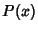andremain Finite at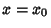, thenis called an Ordinary Point. If eitherordiverges as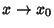, thenis called a singular point. Ifdiverges more quickly than, so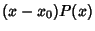approaches Infinity as, ordiverges more quickly thanso that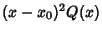goes to Infinity as, thenis called an Irregular Singularity (or Essential Singularity).

Arfken, G. Singular Points.'' §8.4 in Mathematical Methods for Physicists, 3rd ed. Orlando, FL: Academic Press, pp. 451-453 and 461-463, 1985.